## Find the inverse of g(n) = – 3 – 1 Og-1 (n) = -(n-3)-1 9 9-1 (n) = In g-1 (n) = (n + 1)3 + 3 Og-1 (n) = 3+n3<

Question

Find the inverse of g(n) = – 3 – 1
Og-1 (n) = -(n-3)-1
9
9-1 (n) = In
g-1 (n) = (n + 1)3 + 3
Og-1 (n) = 3+n3

in progress 0
1 week 2021-09-05T07:58:43+00:00 1 Answers 0 views 0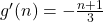Step-by-step explanation:

Given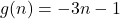Required

Determine the inverseReplace g(n) with y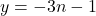Swap positions of x and y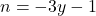Make y the subject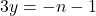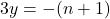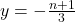Replace y with g'(n)